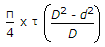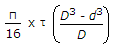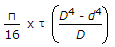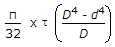# Mechanical Engineering - Strength of Materials

Exercise : Strength of Materials - Section 4
1.
A pressure vessel is said to be a thin shell when it is made of thin sheets.
Agree
Disagree
Explanation:
No answer description is available. Let's discuss.

2.
When a column is subjected to an eccentric load, the stress induced in the column will be
direct stress only
bending stress only
shear stress only
direct and bending stress both
Explanation:
No answer description is available. Let's discuss.

3.
Which of the following statement is wrong?
The deformation of the bar per unit length in the direction of the force is called linear strain.
The Poisson's ratio is the ratio of lateral strain to the linear strain.
The ratio of change in volume to the original volume is called volumetric strain.
The bulk modulus is the ratio of linear stress to the linear strain.
Explanation:
No answer description is available. Let's discuss.

4.
When a shaft is subjected to a twisting moment, every cross-section of the shaft will be under
tensile stress
compressive stress
shear stress
bending stress